# CIE A Level Maths: Pure 3复习笔记8.3.2 Geometry of Complex Multiplication & Division

### Geometry of Complex Multiplication & Division

You now know how conjugation, addition and subtraction affect the geometry of complex numbers on an Argand diagram. Now we can look at the effects of multiplication and division.

#### What do multiplication and division look like on an Argand diagram?

• Let z1 and z2 be two complex numbers
• With moduli r1 and r2 respectively
• And arguments θ1 and θ2 respectively
• To plot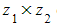on an Argand diagram
• The modulus will be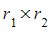• The argument will be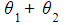• Subtract 2π from the argument if it is not in the range
• To plot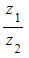on an Argand diagram
• The modulus will be• The argument will be• Add 2π to the argument if it is not in the range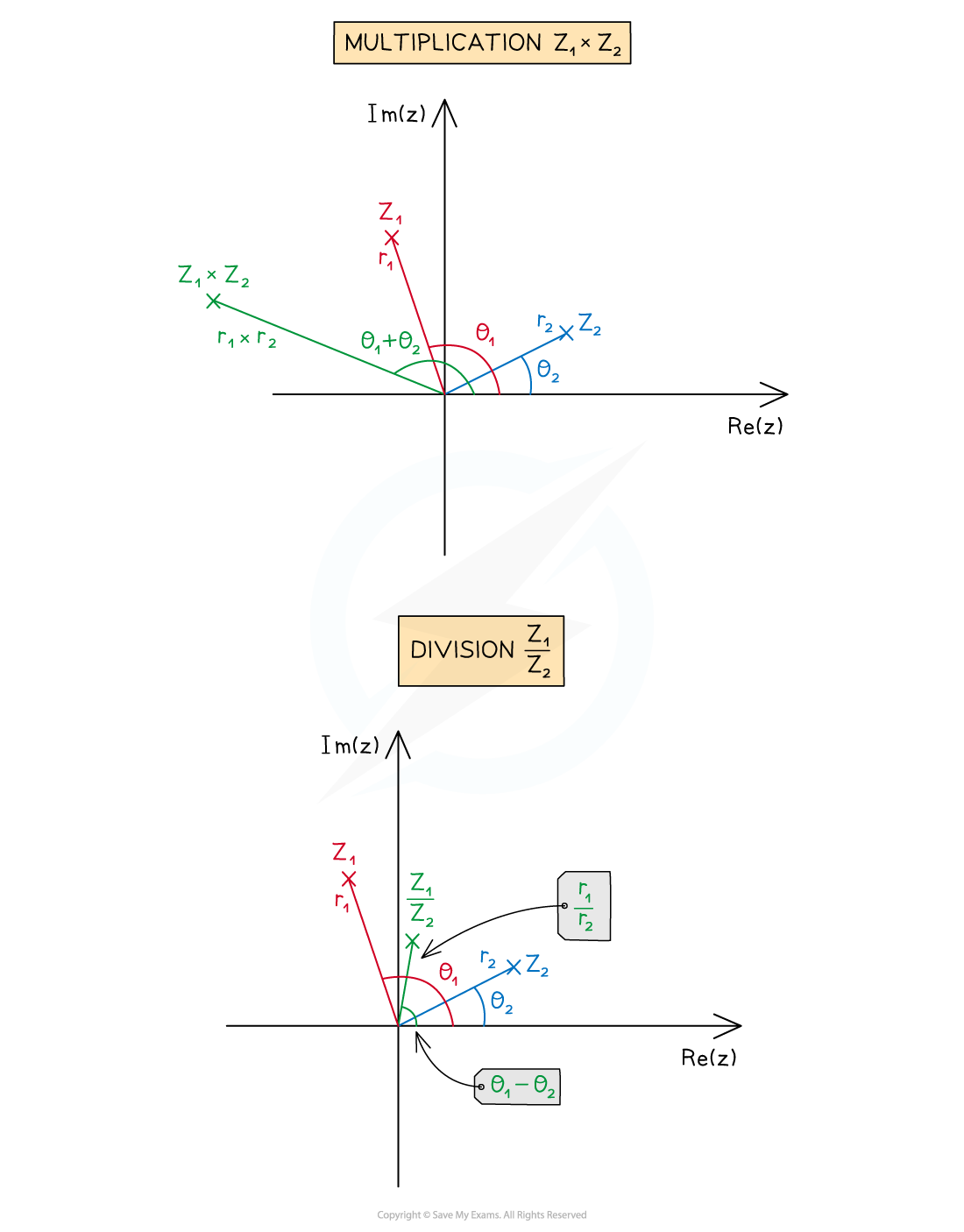#### What are the geometric representations of complex multiplication and division?

• Let w be a given complex number with modulus r and argument θ
• In exponential form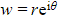• Let z be any complex number represented on an Argand diagram
• Multiplying z by w results in z being:
• Stretched from the origin by a scale factor of r
• If r > 1 then z will move further away from the origin
• If 0 < r < 1 then z will move closer to the origin
• If r = 1 then z will remain the same distance from the origin
• Rotated anti-clockwise about the origin by angle θ
• If θ < 0 then the rotation will be clockwise
• Dividing z by w results in z being:
• Stretched from the origin by a scale factor of
• If r > 1 then z will move closer to the origin
• If 0 < r < 1 then z will further away from the origin
• If r = 1 then z will remain the same distance from the origin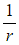• Rotated clockwise about the origin by angle θ
• If θ < 0 then the rotation will be anti-clockwise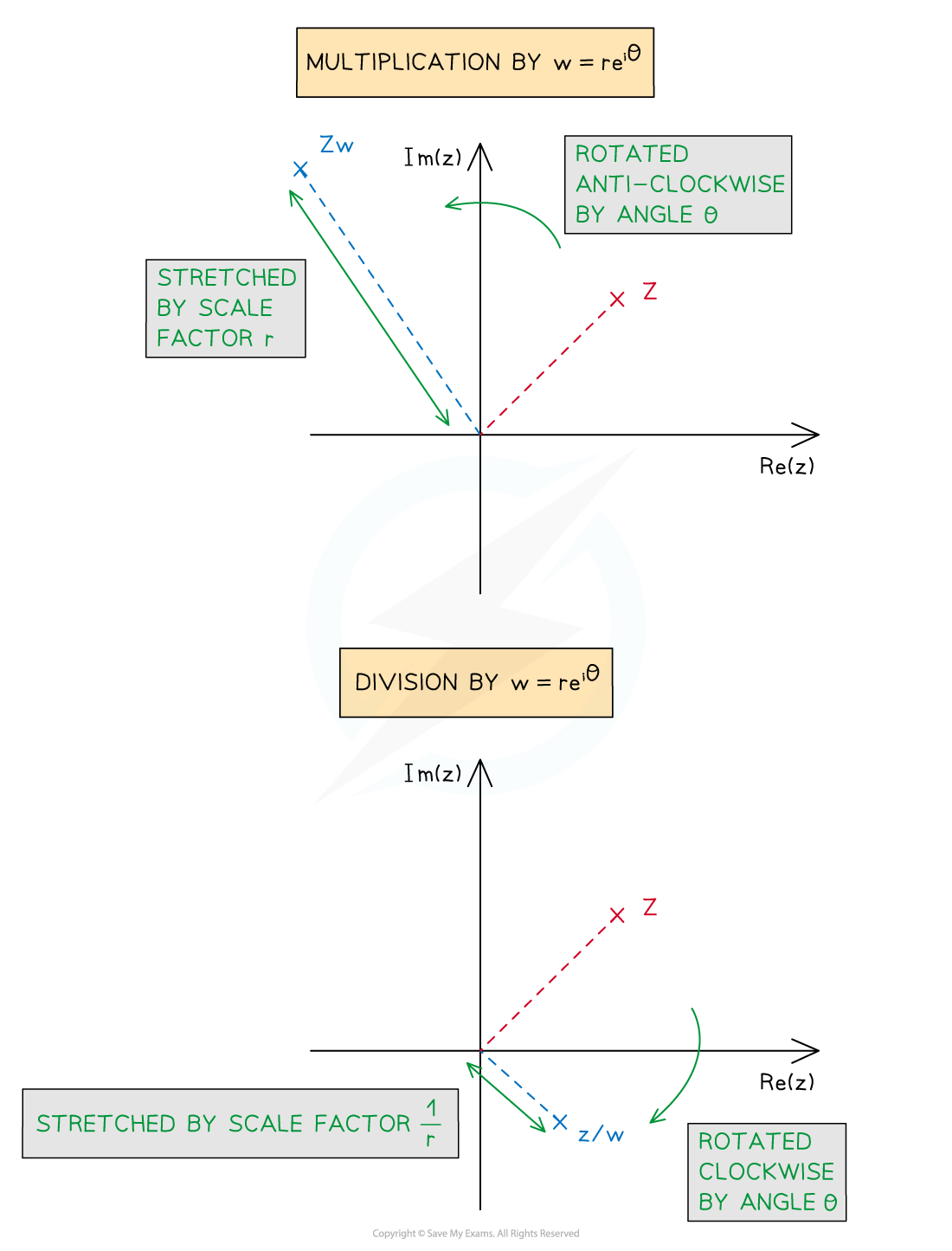#### Worked Example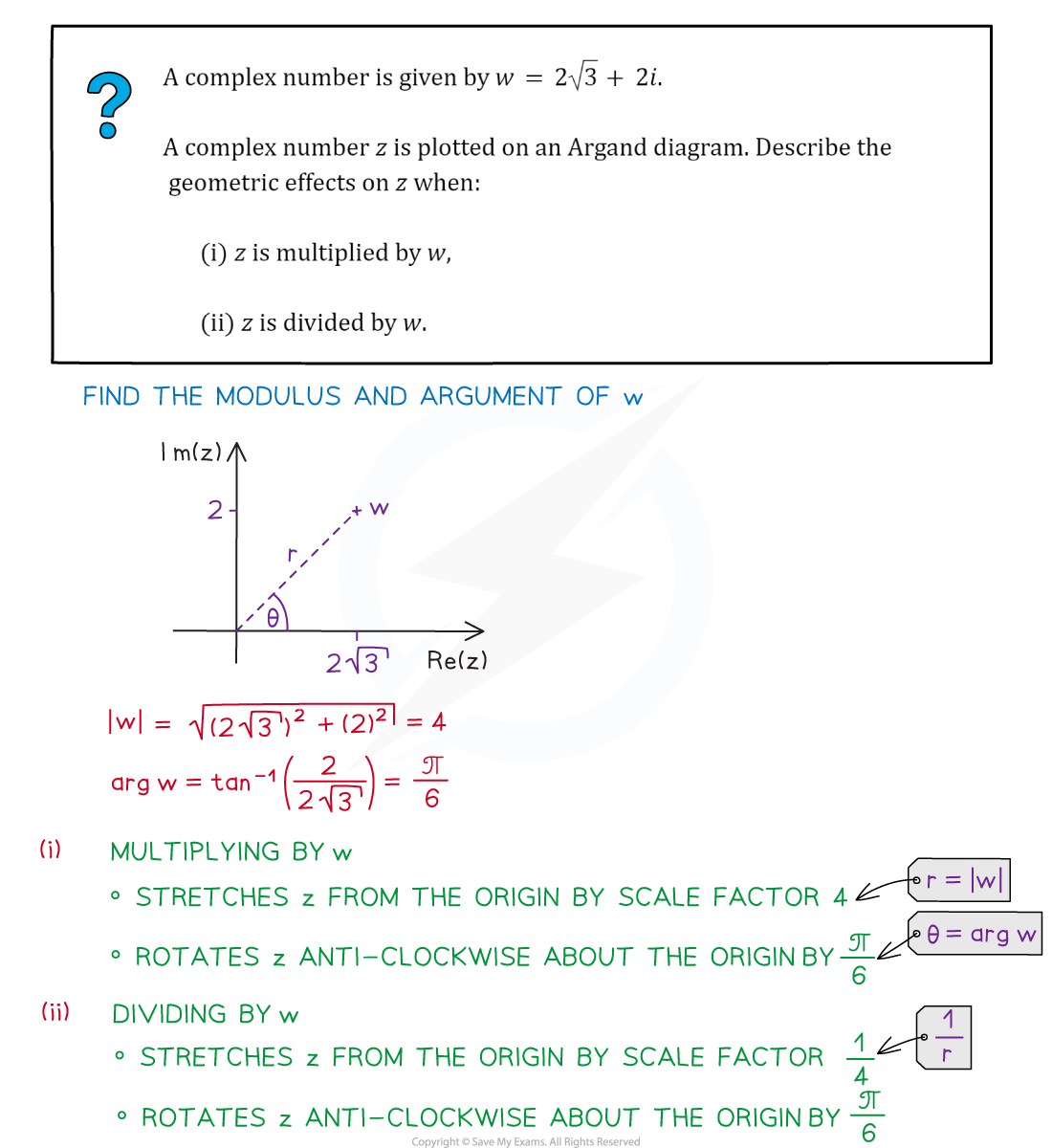#### Exam Tip

• If a complex number is given in Cartesian form, first convert it to polar form or exponential form to find the modulus and argument.